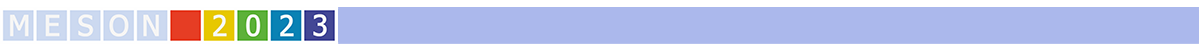#MESON2023

Jun 22 – 27, 2023
Auditorium Maximum
Europe/Warsaw timezone

## Towards a common particle-emitting source in small systems for mesons and baryons with ALICE

Jun 24, 2023, 3:25 PM
20m
Medium lecture hall (A and B) (Auditorium Maximum)

Parallel

### Speaker

Maximilian Korwieser (Technical University of Munich)

### Description

The ALICE Collaboration recently published a plethora of results obtained from femtoscopic measurements, studying the interaction between many exotic combinations of particles, most notably $\mathrm{p}\mbox{--}\Omega$. In general these studies depend on a precise understanding of the particle-emitting source, which is constructed employing the resonance source model (RSM). In the RSM, deviations from a Gaussian source caused by the short-lived resonances are modeled via a Monte Carlo (MC) procedure. The MC procedure is employed to access the convolution of the Gaussian source with the exponential decay functions of the resonances, while taking into account the decay kinematics. For two-particle correlations between baryons ($\mathrm{p}\mbox{--}\mathrm{p}$ and $\mathrm{p}\mbox{--}\Lambda$) the RSM was already validated with great success. The goal of this work is to ascertain whether the RSM can also be applied to constrain the source in the mesonic sector, for example in the case of same charge $\pi\mbox{--}\pi$ (or $\mathrm{K}^{+}\mbox{--}\mathrm{p}$), for which by far the largest contribution from resonances is expected. A differential study of the spatial extension of the source function as a function of transverse mass ($m_{\rm{T}}$) and multiplicity is presented. The results are based on minimum-bias and high-multiplicity pp collisions at $\sqrt{s} = 13 \rm{\,TeV}$ recorded with the ALICE detector. An $m_{\rm{T}}$ scaling behaviour of the source is observed and found to be compatible with previous results in the bayronic sector. This measurement gives confidence for a common source for mesons and baryons in small systems, allowing to employ the RSM to constrain the source for meson$\mbox{--}$baryon and meson$\mbox{--}$meson.

Collaboration ALICE

### Primary author

Maximilian Korwieser (Technical University of Munich)